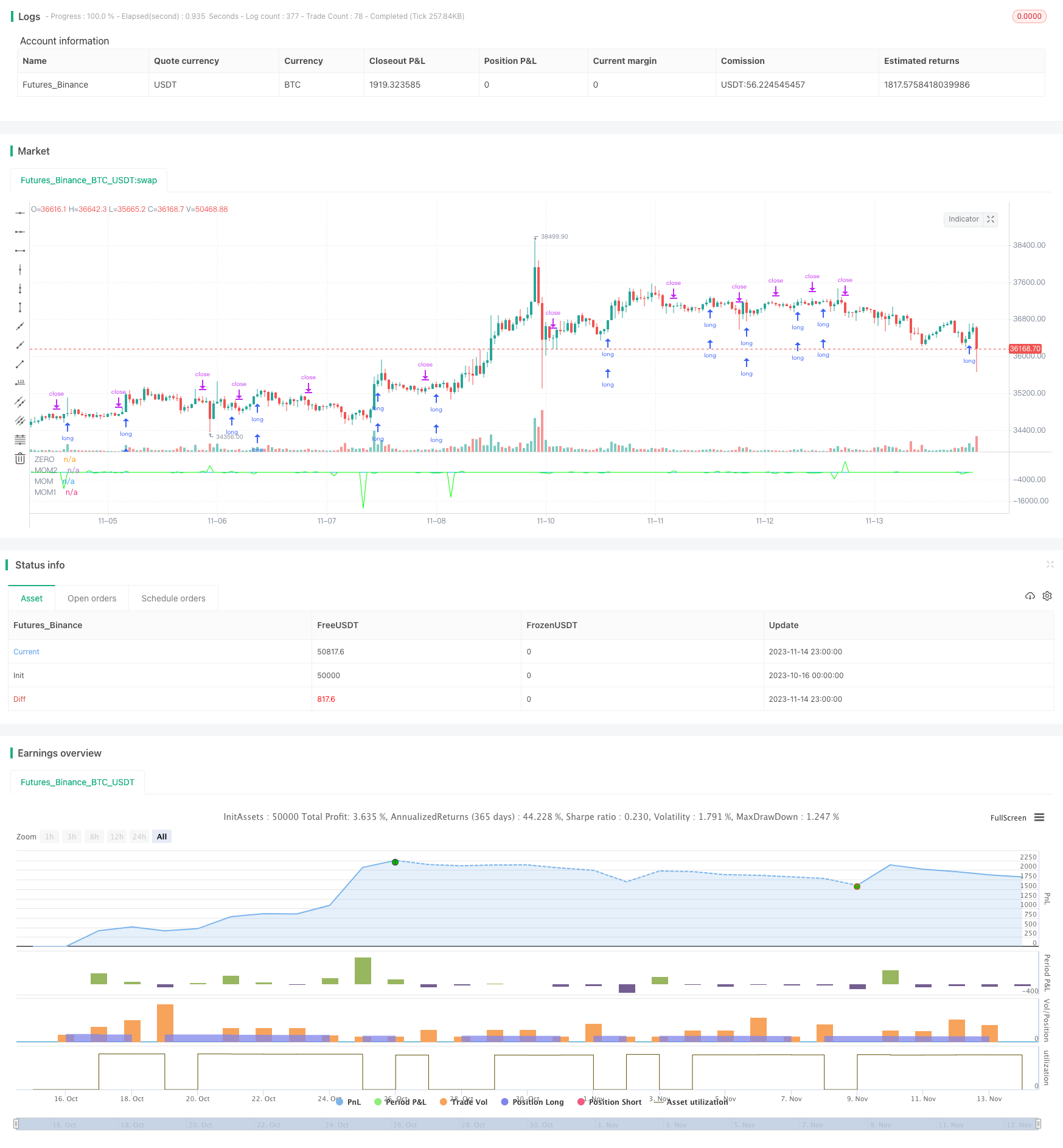# Dual Momentum Breakout Strategy

Author: ChaoZhang, Date: 2023-11-16 17:00:54
Tags:## Overview

This is a dual momentum breakout strategy. It uses two momentum indicators with different parameter settings and generates trading signals when both momentum indicators break through the zero line. The strategy only takes long entries and shorts are only used for exiting positions.

## Strategy Logic

The code first sets strategy properties like order type, commission scheme etc. Then it calculates two momentum indicators:

``````// Momentum settings
****
i_len           = input(defval = 12, title = "Length", minval = 1)
i_src           = input(defval = close, title = "Source")
i_percent       = input(defval = true, title = "Percent?")
i_mom           = input(defval = "MOM2", title = "MOM Choice", options = ["MOM1", "MOM2"])

// Momentum code

mom0 = momentum(i_src, i_len, i_percent)
mom1 = momentum(mom0, 1, i_percent)
mom2 = momentum(i_src, 1, i_percent)

momX = mom1

if i_mom == "MOM2"
momX := mom2
``````

mom0 is the base momentum indicator with length i_len, data source i_src, whether to calculate percentage is decided by i_percent.

mom1 is the momentum indicator with mom0 as data source and length 1.

mom2 is the momentum indicator with original data i_src as source and length 1.

The final momentum indicator momX defaults to mom1, can also choose mom2.

When mom0 and momX both breach above 0 line, go long. When mom0 and momX both breach below 0 line, close position.

1. Using dual momentum indicators with different settings improves signal reliability with double confirmation and fewer false signals.

2. Only taking long entries and using shorts for exits lowers trade frequency and reduces transaction costs.

3. Flexible momentum parameter adjustments suit different market environments.

4. Clean code structure, easy to understand and modify.

## Risks of the Strategy

1. Dual momentum may miss weaker trend signals while reducing false signals.

2. Missing short trade opportunities with only long entries.

4. Insufficient backtest data causes parameter overfitting.

5. Double confirmation reduces but does not eliminate false signals, need to watch for validity in live trading.

## Optimization Directions

1. Test combinations of length and percentage parameters to find optimum.

3. Test different momentum calculations like ROC, RSI etc for better results.

4. Add trend filtering to avoid whipsaw markets.

5. Optimize stop loss for maximum profitability within risk limits.

## Conclusion

This is a typical dual momentum breakout strategy. It uses double confirmation to reduce false signals and only long entries to lower trade frequency. The advantages are simplicity and ease of implementation, with much room for improvements in parameter optimization and risk control. Overall it serves as a reasonable momentum breakout framework but needs market-specific tuning and optimizations for profitable live trading.

```/*backtest
start: 2023-10-16 00:00:00
end: 2023-11-15 00:00:00
period: 1h
basePeriod: 15m
exchanges: [{"eid":"Futures_Binance","currency":"BTC_USDT"}]
*/

//@version=4
strategy("Momentum Long Strategy", overlay = false, precision = 2, initial_capital = 10000, default_qty_value = 10000, default_qty_type = strategy.cash, commission_type = strategy.commission.percent, commission_value = 0, calc_on_every_tick = true)

// There will be no short entries, only exits from long.
strategy.risk.allow_entry_in(strategy.direction.long)

// Calculate start/end date and time condition
startDate  = input(timestamp("2021-01-02T00:00:00"), title = "Start Date", type = input.time)
finishDate = input(timestamp("2021-12-31T00:00:00"), title = "End Date",type = input.time)

time_cond  =true

// Momentum settings

i_len           =       input(defval = 12,      title = "Length",       minval = 1)
i_src           =       input(defval = close,   title = "Source")
i_percent       =       input(defval = true,    title = "Percent?")
i_mom           =       input(defval = "MOM2",  title = "MOM Choice",   options = ["MOM1", "MOM2"])

// Messages for buy and sell

// Momentum code

momentum(seria, length, percent) =>
_mom        =       percent ? ( (seria / seria[length]) - 1) * 100 : seria - seria[length]
_mom

mom0        =       momentum(i_src, i_len, i_percent)
mom1        =       momentum(mom0, 1, i_percent)
mom2        =       momentum(i_src, 1, i_percent)

momX        =       mom1

if i_mom == "MOM2"
momX    :=     mom2

if (mom0 > 0 and momX > 0 and time_cond)
strategy.entry("MomLE", strategy.long, stop = high + syminfo.mintick, comment = "MomLE", alert_message = message_buy)
else
strategy.cancel("MomLE")
if (mom0 < 0 and momX < 0 and time_cond)
strategy.entry("MomExit", strategy.short, stop = low - syminfo.mintick, comment = "MomSE", alert_message = message_sell)
else
strategy.cancel("MomExit")

// Plotting

plot(0, color = #5d606b, title = "ZERO")
plot(mom0, color = #00bcd4, title = "MOM")
plot(mom1, color = #00FF00, title = "MOM1", display = display.none)
plot(mom2, color = #00FF00, title = "MOM2")
```

More